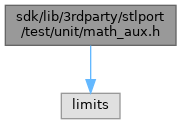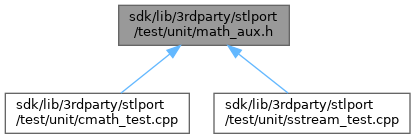ReactOS  0.4.15-dev-5146-g069b08d
math_aux.h File Reference
`#include <limits>`
Include dependency graph for math_aux.h:This graph shows which files directly or indirectly include this file:Go to the source code of this file.

## Macros

#define __STD   std::

## Functions

template<class _Tp >
bool are_equals (_Tp val, _Tp ref)

## ◆ __STD

 #define __STD   std::

Definition at line 8 of file math_aux.h.

## ◆ are_equals()

template<class _Tp >
 bool are_equals ( _Tp val, _Tp ref )

Definition at line 19 of file math_aux.h.

19  {
20  if (val < ref) {
21  return (ref - val) <= __STD numeric_limits<_Tp>::epsilon();
22  }
23  else {
24  return (val - ref) <= __STD numeric_limits<_Tp>::epsilon();
25  }
26 }
Definition: send.c:48
GLuint GLfloat * val
Definition: glext.h:7180
#define __STD
Definition: math_aux.h:8

Referenced by CMathTest::import_checks(), and SstreamTest::init_in().